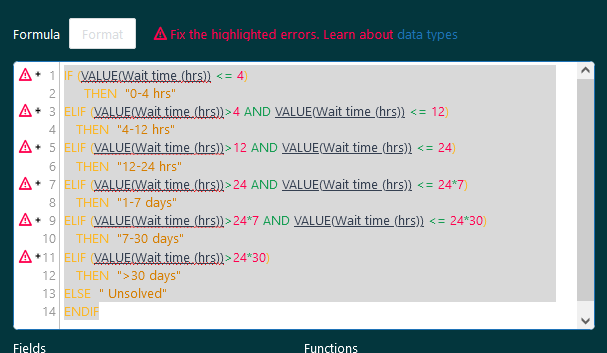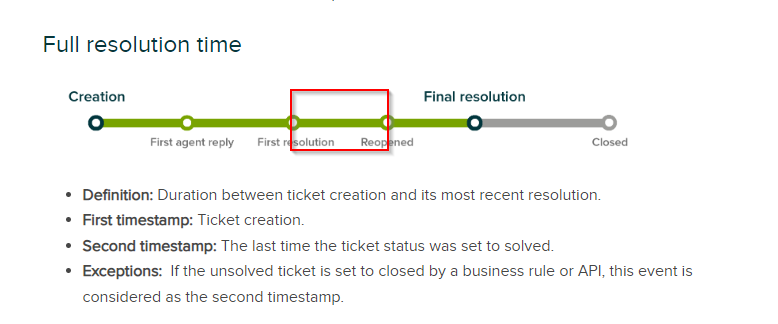• Official comment
Zendesk Product Manager

James Bransden

It is a fair point. We didn't enforce users from using the VALUE aggregator before, but in the majority of the cases, it didn't work well with the calculated metrics and resulted in broken reports or miscalculations.  That's why we advised users only to use it with the database-level metrics as documented here.

Now, the formula validation restricts VALUE aggregator usage with calculated metrics.

You can easily fix your calculations by using the database-level metric, like this:

`IF (VALUE(First reply time - Business hours (min)) < 10*60)THEN [Ticket ID]ENDIF`

Hi Eugene

I can't get this to work with Unsolved tickets age.

We used to have a brackets created with:

`IF (VALUE(Unsolved tickets age (hrs)) <= 5)      THEN  "<5 hrs"ELIF (VALUE(Unsolved tickets age (hrs)) <= 24)    THEN  "5-24 hrs"     ELIF  (VALUE(Unsolved tickets age (hrs)) <= 24*2)   THEN "1-2 days"ELIF  (VALUE(Unsolved tickets age (hrs)) <= 24*4)   THEN "2-4 days"ELIF  (VALUE(Unsolved tickets age (hrs)) <= 24*7)   THEN "4-7 days"ELIF  (VALUE(Unsolved tickets age (hrs)) <= 24*14)   THEN "7-14 days" ELIF  (VALUE(Unsolved tickets age (hrs)) <= 24*30)   THEN "14-30 days" ELIF  (VALUE(Unsolved tickets age (hrs)) > 24*30)    THEN   "30+ days"ELSE  "Unsolved"ENDIF`

But Unsolved tickets age (min) is also an calculated metric so no quick fix.

Right now, I'm using the pre-built metric Unsolved tickets age brackets instead but would like to make my own backets.

Would this really be the solution?:

`IF (DATE_DIFF(NOW(), [Ticket created - Timestamp], "nb_of_hours") <= 5) THEN    "<5 hrs"ELIF (DATE_DIFF(NOW(), [Ticket created - Timestamp], "nb_of_hours") <= 24) THEN    "5-24 hrs"ELIF (DATE_DIFF(NOW(), [Ticket created - Timestamp], "nb_of_hours") <= 24 * 2) THEN    "1-2 days"ELIF (DATE_DIFF(NOW(), [Ticket created - Timestamp], "nb_of_hours") <= 24 * 4) THEN    "2-4 days"ELIF (DATE_DIFF(NOW(), [Ticket created - Timestamp], "nb_of_hours") <= 24 * 7) THEN    "4-7 days"ELIF (DATE_DIFF(NOW(), [Ticket created - Timestamp], "nb_of_hours") <= 24 * 14) THEN    "7-14 days"ELIF (DATE_DIFF(NOW(), [Ticket created - Timestamp], "nb_of_hours") <= 24 * 30) THEN    "14-30 days"ELIF (DATE_DIFF(NOW(), [Ticket created - Timestamp], "nb_of_hours") > 24 * 30) THEN    "30+ days"ELSE    "Unsolved"ENDIF`

Zendesk Product Manager

Troels Lemming Müller, the syntax you provided above is correct. Glad that you found the solution. The results should be identical to the previous version of the formula but if some of the results will feel off let me know here.

Hello Eugine and Zendesk users,

I have a similar problem now counting the number of unsolved tickets based on their current age.

For example to figure out the number of tickets with more than 90 days baklog age I was using this Standard Caluclated Metric:

IF (VALUE(Unsolved tickets age (days)))>90
THEN [Ticket ID]
ENDIF

Then Count that metric in my explore report.

Now I receive the error message regaring VALUE use.

What workaround do you recommand?

Best regards

Zendesk Product Manager

The same workaround that Troels shared above will work for you.

Before

`IF (VALUE(Unsolved tickets age (days)))>90THEN [Ticket ID]ENDIF`

Arter

`IF (DATE_DIFF(NOW(), [Ticket created - Timestamp], "nb_of_hours"))>90THEN [Ticket ID]ENDIF`

Hello,Eugene Orman I belive we have a slight misandrestanding.

I need tos have the number of non closed tickets tthat have a backlog age more than XX hours/days.

So I guess there should be an argument before to pinpoint to the non closed tickets.

`IF (DATE_DIFF(NOW(), [Ticket created - Timestamp], "nb_of_hours"))>90THEN [Ticket ID]ENDIF`

When I use the formula you provided me with with a COUNT I have more than 30k where Is should have 3

Best regards

Hi Franck

In my case I had a filter in place already so I didn't need it, but you can do something like:

`IF ([Ticket status - Unsorted] != "Solved" AND [Ticket status - Unsorted] != "Closed") THEN DATE_DIFF(NOW(), [Ticket created - Timestamp], "nb_of_minutes") ENDIF`

Unfortunately I still have an isuue.

When trying to coount the number of backlog tickets that have more than 70 days:

IF ([Ticket status - Unsorted] != "Solved" AND [Ticket status - Unsorted] != "Closed")

THEN DATE_DIFF(NOW(), [Ticket created - Timestamp], "nb_of_minutes")*60*24*70

ENDIF

I have the same result as the total backlog tickets.

Hi Franck

Unfortunately, I haven't had the need to add something like this as a calculated metric.

So, it might be something like:

`IF ([Ticket status - Unsorted] != "Solved" AND [Ticket status - Unsorted] != "Closed" AND DATE_DIFF(NOW(), [Ticket created - Timestamp], "nb_of_hours") > 24 * 70) THEN    [Ticket ID]ENDIF`

else Eugenes input might be needed.

Hello Troels,

That seem to work fine.

Thank you very much.

Hi Eugene Orman thanks for the assistance on this, seems to working.

Thanks,
James

We use VALUE to nest logic statements in calculated metrics. This lets us create and update calculated metrics by updating a single source calculated metric, instead of going through and updating multiple metrics with the needed changes.

While we can go in and update each calculated metric to fix this, this makes creating and maintaining metrics much harder.

Are there any plans to reintroduce some type of metric handling now that this limitation has been introduced?

For example:

Initial Metric (Returned to Tier 1):

IF ([Changes - Field name]="status"
AND ([Changes - Previous value]="pending"
OR [Changes - Previous value]="solved")
AND [Changes - New value] ="open"
AND [Ticket group] = "Tier 1")
THEN [Ticket ID]
ENDIF

Child Metric:

IF (
VALUE(Returned Tier 1) != NULL
AND [Update - Week of year] > [Ticket created - Week of year]
) THEN [Ticket ID]
ELSE NULL
ENDIF

I have the same issue, can anyone help please? I was using a series of standard calculated metrics like:

`IF(([Chat type - Unsorted]="Inbound")AND(VALUE(Chat wait time (sec)) <= 30))THEN [Chat ID]ENDIF`

We have our own brackets that are different from the built in wait time brackets. Now the formula does not work, I have tried the SUM aggregator and get a green tick, but no results. Not a 0, just nothing i.e. blank.

`IF(([Chat type - Unsorted]="Inbound")AND(SUM(Chat wait time (sec)) <= 30))THEN [Chat ID]ENDIF`

I've tried so many variations of this and I'm at my wit's end.

Thanks,

Steven.

Steven Hampson

What if you try something like:

`IF   ([Chat type - Unsorted]="Inbound"   AND [Chat completion]="Missed"   AND VALUE(Chat no reply time (sec)) <= 30)   THEN    [Chat ID]ELIF   ([Chat type - Unsorted]="Inbound"   AND VALUE(Chat first reply time (sec)) <= 30)THEN    [Chat ID]ENDIF`

We're not using chat, so I can't test it.

Hi Troels,

Thanks for the quick response. Yes this did the trick!! Thank you so much, I appreciate it!

Regards,

Steven.

Hello,

We use this calculated metric (Wait time (hrs)) summing up the time spent only in statuses less than Solved (New+Opem+Pending+On-hold):

`VALUE(Agent wait time (min))/60+VALUE(Requester wait time (min))/60`

And it's used in this formula for defining custom brackets:

`IF (VALUE(Wait time (hrs)) <= 4)      THEN  "0-4 hrs"ELIF (VALUE(Wait time (hrs))>4 AND VALUE(Wait time (hrs)) <= 12)    THEN  "4-12 hrs"    ELIF (VALUE(Wait time (hrs))>12 AND VALUE(Wait time (hrs)) <= 24)    THEN  "12-24 hrs"     ELIF (VALUE(Wait time (hrs))>24 AND VALUE(Wait time (hrs)) <= 24*7)    THEN  "1-7 days"    ELIF (VALUE(Wait time (hrs))>24*7 AND VALUE(Wait time (hrs)) <= 24*30)    THEN  "7-30 days"  ELIF (VALUE(Wait time (hrs))>24*30)    THEN  ">30 days"  ELSE  " Unsolved"ENDIF`

Which displays the errors in the attached capture:Can you please recommend a workaround to define the same formula but with database-level metrics?

Many thanks!

Can you use the metric Full resolution time (min)?

Then you get something like:

`IF (VALUE(Full resolution time (min)) <= 4*60)      THEN  "0-4 hrs"ELIF (VALUE(Full resolution time (min))>4*60 AND VALUE(Full resolution time (min)) <= 12*60)    THEN  "4-12 hrs"    ELIF (VALUE(Full resolution time (min))>12*60 AND VALUE(Full resolution time (min)) <= 24*60)    THEN  "12-24 hrs"     ELIF (VALUE(Full resolution time (min))>24*60 AND VALUE(Full resolution time (min)) <= 24*60*7)    THEN  "1-7 days"    ELIF (VALUE(Full resolution time (min))>24*60*7 AND VALUE(Full resolution time (min)) <= 24*60*30)    THEN  "7-30 days"  ELIF (VALUE(Full resolution time (min))>24*60*30)    THEN  ">30 days"  ELSE  " Unsolved"ENDIF`

Using the full resolution time would be less accurate for our interest than the calculated metric, since we want to capture only the times when a ticket was in statuses less than Solved.

From what I know, the full resolution time includes also the time spent in Solved for tickets that were re-opened... correct me if I am wrong.

We want to exclude the period highlighted in the capture from below:Still looking for alternatives to the calculated metric, with database-level metrics. Hoping that Zendesk experts should be able to help with this, by providing a solution that can easily fix the calculation :)

Otherwise, this change is a downgrade in the service, when no workaround is given to what was possible before.

I will make sure to voice my frustration to our success and account managers that this aggregator was removed for all because there were problems with how it was used by few.

Hi, I'm getting the same error but with a pre-built metric: SLA metric breach time (min). Do you know why that is, or how I can fix it? Trying to create a calculated metric that brackets breach time.

`IF (VALUE(SLA metric breach time (min))/60 <= 4.0) THEN "0-4 hrs"`

Hi,

Same error!

IF (
(
[Ticket status - Unsorted] = "Solved"
OR  [Ticket status - Unsorted] = "Closed"
)
AND [Priority Value] != "Urgent 1"
) THEN
IF (
(
[Tier [formula]] = "Tier 1"
AND
VALUE(First resolution time - Business hours (hrs))
- VALUE(Agent wait time - Business hours (hrs))
<= 48
)
OR (
(
[Tier [formula]] = "Tier 2"
OR [Tier [formula]] = "Tier 3"
)
AND (
(
VALUE(First resolution time - Business hours (hrs))
- VALUE(Agent wait time - Business hours (hrs))
<= 72
AND [Priority Value] = "High 2"
)
OR (
VALUE(First resolution time - Business hours (hrs))
- VALUE(Agent wait time - Business hours (hrs))
<= 168
AND [Priority Value] = "Normal/Low 3"
)
)
)
) THEN
1
ENDIF
ENDIF

Hi All,

The above issue is resolved!

Please help on the below metric, here we are trying to get the when the group assignee is changed to another group and how much time spent on previous group assignment.

Where I have calculated metric

IF
ATTRIBUTE_FIX(D_COUNT(Assignment Timestamps - T3 HR Tech), [Ticket ID]) > 0
THEN
IF
[Ticket group] = "T3 HR Tech"
THEN
IF
ATTRIBUTE_FIX(D_COUNT(Assignment Timestamps - T3 HR Tech), [Ticket ID]) > 1
THEN
VALUE(Time Since Last Group Assign (min)) + ATTRIBUTE_FIX(SUM(Time spent in prev group - T3 HR Tech (min)), [Ticket ID])
ELSE
VALUE(Time Since Last Group Assign (min))
ENDIF
ELSE
ATTRIBUTE_FIX(SUM(Time spent in prev group - T3 HR Tech (min)), [Ticket ID])
ENDIF
ENDIF

The calculation for Assignment Timestamps - T3 HR Tech
IF
[Update ticket group] = "T3 HR Tech" AND [Changes - Field name] = "group_id"
THEN
[Update - Timestamp]
ENDIF

The calculation for Time Since Last Group Assign (min)

IF
IN([Ticket status - Unsorted], ARRAY("Solved", "Closed"))
THEN
DATE_DIFF([Ticket solved - Timestamp], DATE([Latest Group Assignment - Timestamp]), "nb_of_minutes")
ELSE
DATE_DIFF(NOW(), DATE([Latest Group Assignment - Timestamp]), "nb_of_minutes")
ENDIF

Zendesk Product Manager

Adrian Mitrea, You can simply replace the nested metric VALUE(Wait time (hrs)) with its formula VALUE(Agent wait time (min))+VALUE(Requester wait time (min)). And perhaps slightly clean the final formula, to get something like this:

`IF (VALUE(Agent wait time (min))+VALUE(Requester wait time (min)) <= 4*60)      THEN  "0-4 hrs"ELIF (VALUE(Agent wait time (min))+VALUE(Requester wait time (min))>4*60 AND VALUE(Agent wait time (min))+VALUE(Requester wait time (min)) <= 12*60)    THEN  "4-12 hrs"    ELIF (VALUE(Agent wait time (min))+VALUE(Requester wait time (min))>12*60 AND VALUE(Agent wait time (min))+VALUE(Requester wait time (min)) <= 24*60)    THEN  "12-24 hrs"     ELIF (VALUE(Agent wait time (min))+VALUE(Requester wait time (min))>24*60 AND VALUE(Agent wait time (min))+VALUE(Requester wait time (min)) <= 24*60)    THEN  "1-7 days"    ELIF (VALUE(Agent wait time (min))+VALUE(Requester wait time (min))>24*7*60 AND VALUE(Agent wait time (min))+VALUE(Requester wait time (min)) <= 24*30*60)    THEN  "7-30 days"  ELIF (VALUE(Agent wait time (min))+VALUE(Requester wait time (min))>24*30*60)    THEN  ">30 days"  ELSE  " Unsolved"ENDIF`